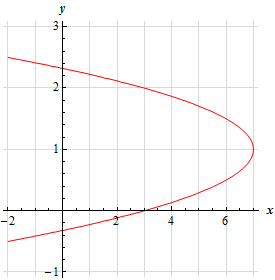Paul's Online Notes
Home / Calculus I / Review / Common Graphs
Show Mobile Notice Show All Notes Hide All Notes
Mobile Notice
You appear to be on a device with a "narrow" screen width (i.e. you are probably on a mobile phone). Due to the nature of the mathematics on this site it is best views in landscape mode. If your device is not in landscape mode many of the equations will run off the side of your device (should be able to scroll to see them) and some of the menu items will be cut off due to the narrow screen width.

### Section 1.10 : Common Graphs

12. Without using a graphing calculator sketch the graph of $$f\left( y \right) = - 4{y^2} + 8y + 3$$.

Hint : Recall that the graph of $$f\left( y \right) = a{y^2} + by + c$$ is the graph of a parabola with vertex $$\left( {f\left( { - \frac{b}{{2a}}} \right), - \frac{b}{{2a}}} \right)$$ that opens towards the right if $$a > 0$$ and towards the left if $$a < 0$$ and $$x$$-intercept at $$\left( {c,0} \right)$$.
Show Solution

We know that the graph of $$f\left( y \right) = a{y^2} + by + c$$ will be a parabola that opens towards the right if $$a > 0$$ and opens towards the left if $$a < 0$$. We also know that its vertex is at,

$\left( {f\left( { - \frac{b}{{2a}}} \right), - \frac{b}{{2a}}} \right)$

The $$x$$-intercept of the parabola is the point $$\left( {f\left( 0 \right),0} \right) = \left( {c,0} \right)$$ and the $$y$$-intercepts (if any) are found by solving $$f\left( y \right) = 0$$

So, or our case we know we have a parabola that opens towards the left and that its vertex is at,

$\left( {f\left( { - \frac{8}{{2\left( { - 4} \right)}}} \right), - \frac{8}{{2\left( { - 4} \right)}}} \right) = \left( {f\left( 1 \right),1} \right) = \left( {7,1} \right)$

We can also see that the $$x$$-intercept is $$\left( {3,0} \right)$$.

To find the $$y$$-intercepts all we need to do is solve : $$- 4{y^2} + 8y + 3 = 0$$.

$y = \frac{{ - 8 \pm \sqrt {{8^2} - 4\left( { - 4} \right)\left( 3 \right)} }}{{2\left( { - 4} \right)}} = \frac{{ - 8 \pm \sqrt {112} }}{{ - 8}} = \frac{{ - 8 \pm 4\sqrt 7 }}{{ - 8}} = \frac{{2 \pm \sqrt 7 }}{2} = - 0.3229,\,\,\,2.3229$

So, the two $$y$$-intercepts are : $$\left( {0, - 0.3229} \right)$$ and $$\left( {0,2.3229} \right)$$.

Here is a sketch of this parabola.